# High School Physics : Heat

## Example Questions

← Previous 1 3

### Example Question #1 : Heat

A microwave heats up a cold piece of pizza. What type of heat transfer is this?

Conduction

There is no heat transfer

Induction

Convection

Explanation:

Microwaves use radiation as the method of heat transfer. Radiation refers to heat transfer via electromagnetic waves, such as microwaves.

Conduction is heat transfer via direct contact between two objects. Convection is heat transfer via the movement of surrounding fluids. Induction is not a type of heat transfer.

### Example Question #2 : Heat

A pot of water boils. What type of heat transfer occurs within the pot?

Conduction

Induction

There is no heat transfer

Convection

Convection

Explanation:

Convection is heat transfer via the movement of surrounding fluids. Though conduction may be used to heat the pot, and in turn to heat the water molecules in contact with the edge of the pot, convection occurs inside the pot to transfer heat between the water molecules. This allows the water to heat evenly. Without convection occurring within the pot, the water at the outer edges would be much hotter than the water at the center.

Radiation refers to heat transfer via electromagnetic waves. Conduction is heat transfer via direct contact between two objects. Induction is not a type of heat transfer.

### Example Question #3 : Heat

A piece of ice is placed on a hot surface and begins to melt, but its temperature does not increase. What type of heat transfer is this?

Conduction

Induction

Convection

There is no heat transfer

Conduction

Explanation:

Heat is a form of energy, and can be used to alter the energy of chemical interactions. Ice, a solid, will be in a lower energy state than water, a liquid. The addition of heat energy allows for the increase in phase energy from solid to liquid. The energy addition is converted to a different form; thus, the temperature does not change. Conduction is heat transfer via direct contact between two objects, which describes the transfer between the hot surface and the ice.

Radiation refers to heat transfer via electromagnetic waves, such as microwaves. Convection is heat transfer via the movement of surrounding fluids. Induction is not a type of heat transfer.

### Example Question #1 : Understanding The Concept Of Energy Transfer

A piece of tin sitting on a stove top becomes very hot. What type of heat transfer was involved?

There is no heat transfer

Induction

Convection

Conduction

Conduction

Explanation:

Conduction is heat transfer via direct contact between two objects. As the piece of tin is in direct contact with the stove, heat can freely flow from the stove into the tin, causing it to become hotter.

Radiation refers to heat transfer via electromagnetic waves, such as microwaves. Convection is heat transfer via the movement of surrounding fluids. Induction is not a type of heat transfer.

### Example Question #5 : Heat

On a cold day, the temperature often feels colder due to a wind chill. This is an example of what type of phenomenon?

Induction

Conduction

Convection

None of these

Convection

Explanation:

On a cold day, the air temperature is less than your body temperature. As cold wind comes in contact with your body, heat is transferred from your body to the air moving past, making you colder. This type of heat transfer is known as convection, in which heat is transferred from an object (your body) to a fluid medium (the air).

Conduction is heat transfer due to direct contact between objects. Radiation is heat transfer due to electromagnetic waves. Induction is not a form of heat transfer.

### Example Question #6 : Heat

A piece of bread is burned on one side and uncooked on the other after being heated in a toaster. What was the most likely form of heat transfer to cause the burn?

Conduction

Induction

Convection

Conduction

Explanation:

Conduction is a form of heat transfer requiring direct contact between two objects. In the question, the burned side of the bread was likely in direct contact with the heat source, while the other was only in contact with hot air. The burned side is heated by conduction, while the uncooked side is heated by convection.

Convection is the transfer of heat through a fluid medium, namely a liquid or gas. Radiation is the transfer of heat through electromagnetic waves.

### Example Question #7 : Heat

Which of the following methods of heat transfer requires the two objects to be touching?

Induction

Conduction

Thermodynamic discharge

Convection

Conduction

Explanation:

Conduction is the form of heat transfer that requires direct contact between two objects.

Radiation is heat transfer via electromagnetic radiation. Convection uses a fluid medium, such as air or water, for heat transfer. Induction and thermodynamic discharge are not recognized types of heat transfer.

### Example Question #1 : Understanding The Concept Of Energy Transfer

A marshmallow on a stick is placed above a fire, but not touching the fire. The marshmallow heats up and softens. How is the marshmallow being heated?

I. Conduction

II. Convection

II only

I, II, and III

II and III

I and II

III only

II and III

Explanation:

Conduction occurs when heat is transferred by direct contact between two objects. Convection occurs when heat is transferred via contact between a fluid and an object. Radiation is heat transfer via electromagnetic radiation.

In this question, the fire is a source of heat and electromagnetic radiation. When the radiation from the fire impacts the marshmallow, it is being heated by radiation. The fire is also heating the surrounding air, creating fluid currents. The heated air is also transferring energy to the marshmallow, heating by convection.

There is no conduction in this example.

### Example Question #9 : Heat

A Carnot Cycle operates using a heat reservoir at a temperature of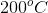and a cold reservoir at a temperature of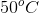. What is the operating efficiency,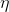, of this cycle, to the nearest percent?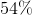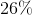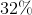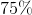Explanation:

The efficiency of a Carnot Cycle is defined by:In this calculation, temperatures are given in Kelvin. Since Celsius and Kelvin exist in a one-to-one ratio, a conversion is unnecessary unless desired. We will perform the conversion, since most classes will advise it.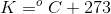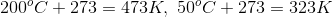Using these values, we can calculate the efficiency: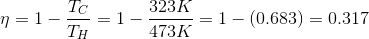Convert this value to a percentage: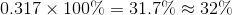### Example Question #1 : Understanding Heat And Temperature

The temperature of an ideal gas is raised from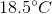to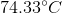. If the volume remains constant, what was its initial pressure if the final pressure is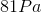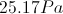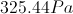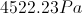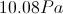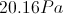Explanation:

For this problem, use Gay-Lussac's law to set up a direct proportion between pressure and temperature. Note that this law only applies when volume is constant.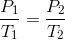Plug in our given values and solve for the initial pressure.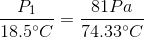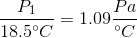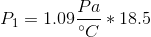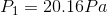← Previous 1 3